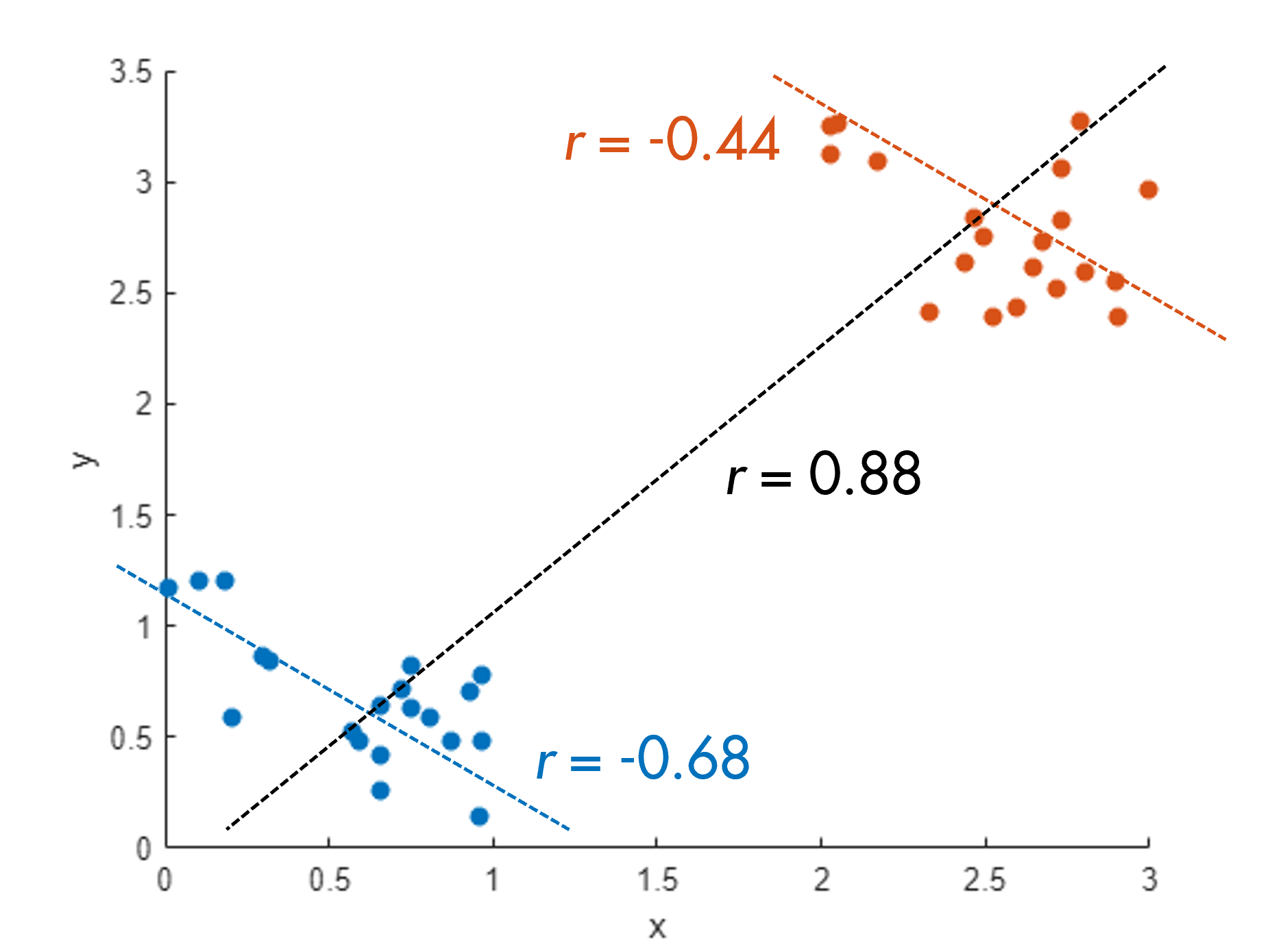# Problem 55225. Simpson's Paradox - Calculate correlation coefficients for groups of data

Simpson's Paradox is a statistical phenomenon where groups of data can have a characteristic while the whole data set together has the opposite characteristic. In the example below, both groups have a negative correlation between x and y, but collectively there is a positive correlation.Write a function that takes three vectors as input: x, y, and g. The vector g will contain only the values 1 and 2. The function should return three outputs. These outputs are the Pearson correlation coefficients for three different groupings of the data, which are: (1) for all x and y, (2) x and y corresponding to elements where g has the value 1, (3) x and y for which g is 2.
[c,c1,c2] = groupcorr(x,y,g)
c =
0.8800
c1 =
-0.6800
c2 =
-0.4396

### Solution Stats

47.99% Correct | 52.01% Incorrect
Last Solution submitted on Dec 02, 2023

### Community Treasure Hunt

Find the treasures in MATLAB Central and discover how the community can help you!

Start Hunting!